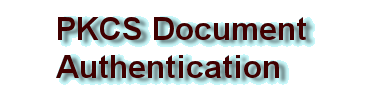| EscapeE Home  | About Us  | Search  | Next  |Document authentication
 Public-key cryptography - mathematicsAsymmetric ciphers are used to allow to communicate securely without using a shared secret key. A "public" and a "private" key are derived from a pair of very large prime numbers. However, it is possible to demonstrate the formula using small numbers. RedTitan use RSA (named for its inventors: Ron Rivest, Adi Shamir, and Leonard Adleman) cryptography. It is a good idea to choose a popular algorithm because there will be a choice of authentication mechanisms on the destination computer and the prudent user can get a second opinion. In some jurisdictions, RSA signatures have a legal status and may be used to validate a document in much the same way as a handwritten signature.NextOriginally postulated by Cliffords Cocks at the British GCHQ the trapdoor function that became the basis for RSA relies on the difficulty of factoring the multiple to two very large prime numbers. The formula is very simple.
• P is any prime number

• Q is any prime number

• E (public exponent) is chosen to be a whole number greater than 1 but less than P*Q where E and (P - 1) * (Q - 1) are relatively prime.
i.e. they have no prime factors in common.

• D (private exponent) is chosen to be any whole number such that the formula (D * E - 1) / (P - 1) * (Q - 1) is a whole positive integer.
e.g. any prime exceeding max(P, Q) (and less than (P*Q) ) would be a valid choice for D.
To encrypt a number T where T <= P * Q apply the formula
(T E) mod ( P * Q )
To decrypt a number C that was produced from the encryption formula apply.
(CD) mod (P * Q)
For example,
P = 11
Q = 3
E = 3
D = 7
To encrypt the number calculate (53 mod (11 *3))  ==  (5 * 5 * 5 mod 33) == (125 mod 33) == 26

To decrypt the number 26 calculate (267 mod (11 * 3 ))
== (26*26* 26 *26*26*26*26 mod 33) ==(8031810176 mod 33)== 5

The public key is the pair of numbers (P * Q, E) or (33, 3) and the private key is (D) or the number 7. In fact, these are the smallest numbers we can choose and the formula is very easy to calculate by hand. This example could not be used as a very good cipher - many numbers in the range (0 to 33) encrypt to exactly the same value. Although the modulus (P *Q) is never published as a pair of prime numbers, it is easy to guess that 33 can only factored to 3 and 11 and with this information it is possible to deduce the private key.

Picking the numerically larger value to be d allows implementing code to take advantage of certain computational improvements to be had in the encryption/decryption arithmetic if the original values of p and q are available, as they could be for the "private" key

RSA becomes very difficult indeed to crack when much larger pair of prime numbers are chosen. Blocks of 128 bytes at time can be encrypted using a 1024 bit key. In this case, two prime 512 bit prime numbers are used to calculate the modulus. Given the modulus from a public key, a brute force scan for a suitable pair of primes will take more time than is left in the universe (It is possible that the CIA know better)